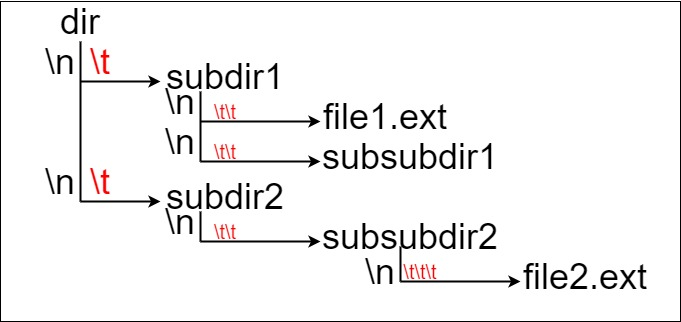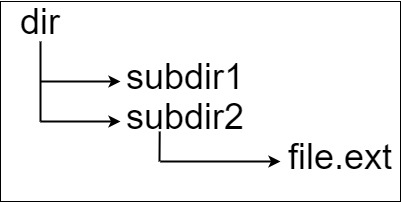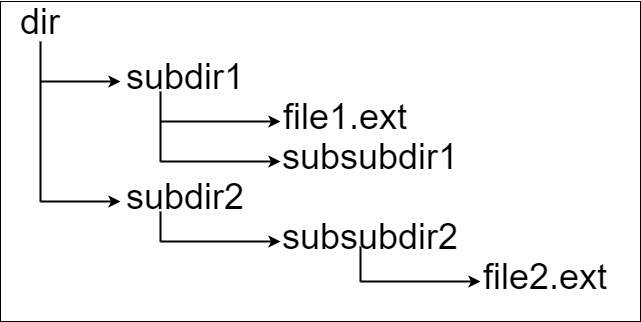## Algorithm

Problem Name: 388. Longest Absolute File Path

Suppose we have a file system that stores both files and directories. An example of one system is represented in the following picture:Here, we have `dir` as the only directory in the root. `dir` contains two subdirectories, `subdir1` and `subdir2`. `subdir1` contains a file `file1.ext` and subdirectory `subsubdir1`. `subdir2` contains a subdirectory `subsubdir2`, which contains a file `file2.ext`.

In text form, it looks like this (with ⟶ representing the tab character):

```dir
⟶ subdir1
⟶ ⟶ file1.ext
⟶ ⟶ subsubdir1
⟶ subdir2
⟶ ⟶ subsubdir2
⟶ ⟶ ⟶ file2.ext
```

If we were to write this representation in code, it will look like this: `"dir\n\tsubdir1\n\t\tfile1.ext\n\t\tsubsubdir1\n\tsubdir2\n\t\tsubsubdir2\n\t\t\tfile2.ext"`. Note that the `'\n'` and `'\t'` are the new-line and tab characters.

Every file and directory has a unique absolute path in the file system, which is the order of directories that must be opened to reach the file/directory itself, all concatenated by `'/'s`. Using the above example, the absolute path to `file2.ext` is `"dir/subdir2/subsubdir2/file2.ext"`. Each directory name consists of letters, digits, and/or spaces. Each file name is of the form `name.extension`, where `name` and `extension` consist of letters, digits, and/or spaces.

Given a string `input` representing the file system in the explained format, return the length of the longest absolute path to a file in the abstracted file system. If there is no file in the system, return `0`.

Note that the testcases are generated such that the file system is valid and no file or directory name has length 0.

Example 1:```Input: input = "dir\n\tsubdir1\n\tsubdir2\n\t\tfile.ext"
Output: 20
Explanation: We have only one file, and the absolute path is "dir/subdir2/file.ext" of length 20.
```

Example 2:```Input: input = "dir\n\tsubdir1\n\t\tfile1.ext\n\t\tsubsubdir1\n\tsubdir2\n\t\tsubsubdir2\n\t\t\tfile2.ext"
Output: 32
Explanation: We have two files:
"dir/subdir1/file1.ext" of length 21
"dir/subdir2/subsubdir2/file2.ext" of length 32.
We return 32 since it is the longest absolute path to a file.
```

Example 3:

```Input: input = "a"
Output: 0
Explanation: We do not have any files, just a single directory named "a".
```

Constraints:

• `1 <= input.length <= 104`
• `input` may contain lowercase or uppercase English letters, a new line character `'\n'`, a tab character `'\t'`, a dot `'.'`, a space `' '`, and digits.
• All file and directory names have positive length.

## Code Examples

### #1 Code Example with C Programming

```Code - C Programming```

``````
int lengthLongestPath(char* input) {
int stack;
int sz = 100, sp = 0;
int l = 0, n = 0;
int isfile = 0, nextline = 1;
int max = 0;

if (!input) return 0;

stack = 0;

while (*input) {
while (*input == '\t') {
n++; input ++;
}
while (*input && *input != '\n') {
if (*input == '.') isfile = 1;
l++; input ++;
}
if (isfile) {
l += stack[n];
max = max < l ? l : max;
isfile = 0;
} else {
l ++; // slash
stack[n + 1] = stack[n] + l;
}
n = 0; l = 0;
input ++;
}
//if (isfile && max < l) {
//    max = l;
//}
return max;
}
``````
Copy The Code &

Input

cmd
input = "dir\n\tsubdir1\n\tsubdir2\n\t\tfile.ext

Output

cmd
20

### #2 Code Example with Java Programming

```Code - Java Programming```

``````
class Solution {
public int lengthLongestPath(String input) {
int maxLen = 0;
int currLevel = 1;
boolean isFile = false;
int currLen = 0;
Map map = new HashMap<>();
map.put(0, 0);
int idx = 0;
int n = input.length();
while (idx < n) {
while (idx < n && input.charAt(idx) == '\t') {
idx++;
currLevel++;
}
while (idx < n && input.charAt(idx) != '\n') {
if (input.charAt(idx) == '.') {
isFile = true;
}
currLen++;
idx++;
}
if (isFile) {
maxLen = Math.max(maxLen, map.get(currLevel - 1) + currLen);
}
else {
map.put(currLevel, map.get(currLevel - 1) + 1 + currLen);
}
currLen = 0;
currLevel = 1;
isFile = false;
idx++;
}
return maxLen;
}
}
``````
Copy The Code &

Input

cmd
input = "dir\n\tsubdir1\n\tsubdir2\n\t\tfile.ext

Output

cmd
20

### #3 Code Example with Javascript Programming

```Code - Javascript Programming```

``````
const lengthLongestPath = function(input) {
const stack = []
return input.split('\n').reduce((max, p) => {
const level = p.lastIndexOf('\t') + 1
stack[level] = p.length - level + (level ? stack[level - 1] : 0)
return p.indexOf('.') === -1 ? max : Math.max(max, stack[level] + level)
}, 0)
}
``````
Copy The Code &

Input

cmd
input = "dir\n\tsubdir1\n\t\tfile1.ext\n\t\tsubsubdir1\n\tsubdir2\n\t\tsubsubdir2\n\t\t\tfile2.ext"

Output

cmd
32

### #4 Code Example with Python Programming

```Code - Python Programming```

``````
class Solution:
def lengthLongestPath(self, input: str) -> int:
maxlen = 0
pathlen = {0: 0}
for line in input.splitlines():
name = line.lstrip('\u005Ct')
depth = len(line) - len(name)
if '.' in name:
maxlen = max(maxlen, pathlen[depth] + len(name))
else:
pathlen[depth + 1] = pathlen[depth] + len(name) + 1
return maxlen
``````
Copy The Code &

Input

cmd
input = "dir\n\tsubdir1\n\t\tfile1.ext\n\t\tsubsubdir1\n\tsubdir2\n\t\tsubsubdir2\n\t\t\tfile2.ext"

Output

cmd
32